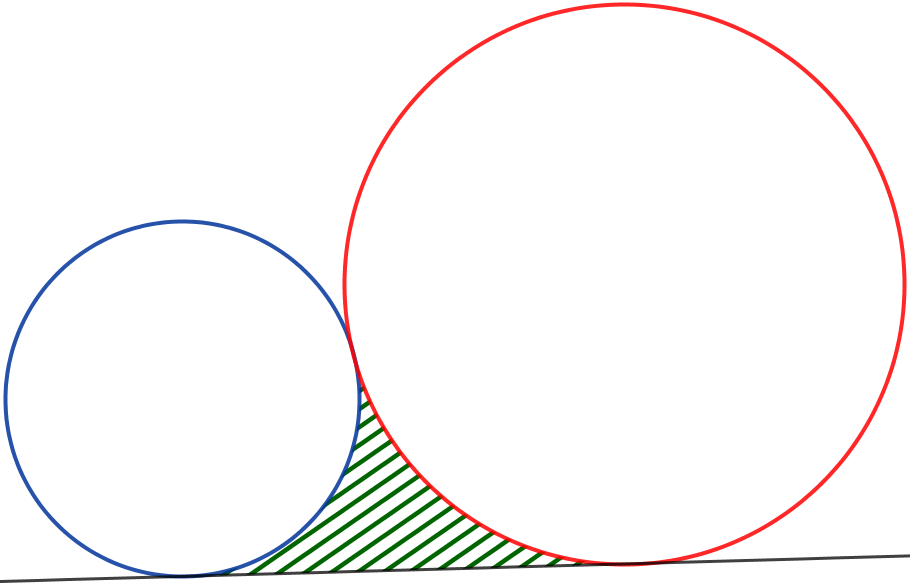# Rollin' Stones

Geometry Level 5Find the area of the hatched region, if the radii of the two circles in the figure above are $1$ and $\sqrt{162+27\sqrt{162+27\sqrt{\cdots}}}$.

If your answer is in the form of $a\sqrt{b}-\frac{c}{2b}\pi$ for some positive pairwise coprime integers $a, b, c$. Find $a+b+c$.

×

Problem Loading...

Note Loading...

Set Loading...